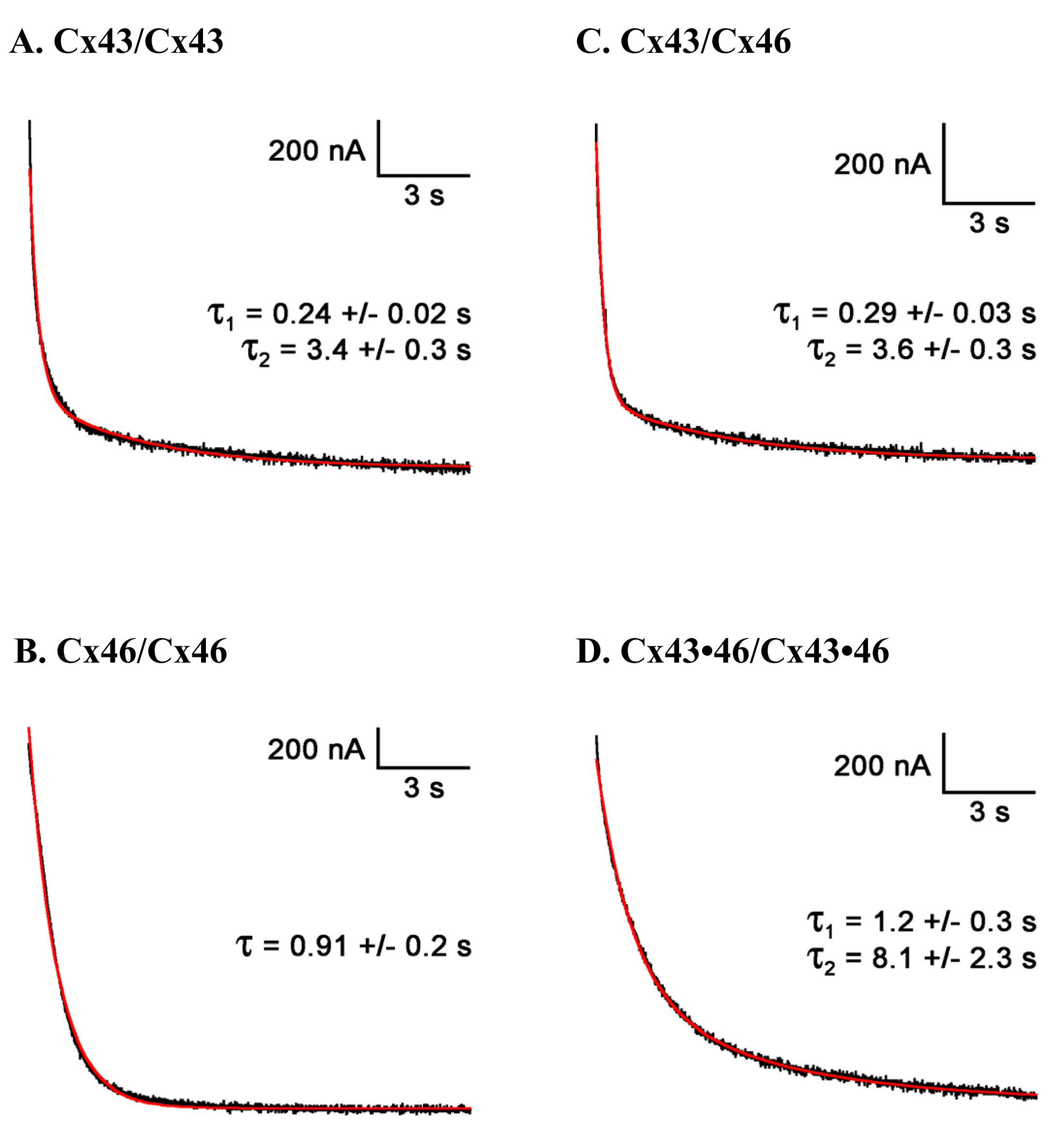Figure 6 of Hoang, Mol Vis 2010; 16:1343-1352.

Figure 6. The kinetics of the voltage-dependent closure of gap junctional channels for homotypic (A, B), heterotypic (C), and heteromeric (D) pairs of RPE Cxs expressed in Xenopus oocytes. For homotypic pairs of Cx43/Cx43 (A), the junctional current was best fit with a second-order exponential decay, whereas homotypic Cx46 (B) could be described by a single exponential function with averaged time constants shown above (n=5 and n=3, respectively). Gating kinetics for the heterotypic Cx43/Cx46 gap junctional channel (C) also required two exponential components (n=6). Heteromeric gap junctions (Cx43•46/Cx43•46) exhibited significantly slower gating kinetics, requiring a second-order exponential function, with the time constants shown in D, above (n=10).